# How to solve a 3x3x3

Aug 11, 2021

If you would prefer a video tutorial then search ‘speedcubing.org how to solve a 3x3x3’ on youtube

Step 1: buy a good cubeThe old cube you have lying around is probably not very good and it is highly recommended that you buy a better cube before attempting to solve it.

Recommended  3x3: Meilong 3x3

Step 2: learn the notation

You have to understand what the following notation means (each letter represents a turn of the face shown in the arrow and the arrow shows the direction):Step 3: solve the crossFind an edge of your chosen cross colour and then bring it down into the bottom face.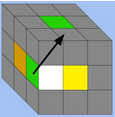Match the edge on the bottom layer with the centre of the same colour.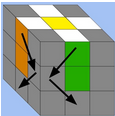Rotate the white edges to the white centre, making sure that the other edge colour matches the centre.

Step 4: solve the first layer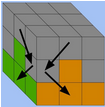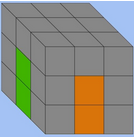Rotate the cube so that the cross you have solved is at the bottom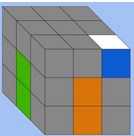Hold the corner you want to go into the first layer directly above where you want it to go

And then repeat this algorithm up to 5 times and it will go in place: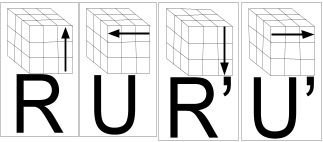Then repeat this for each corner until you have the whole first layer completed

Step 5: solve the second layerHold the edge above where you want it to go (with the edge colour matching the centre), if you need to move it to the right as above do the following algorithm: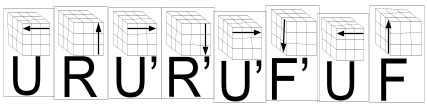Alternatively, if you need to move it to the left, as above, then do this algorithm: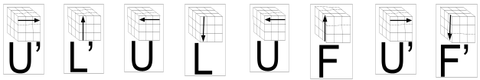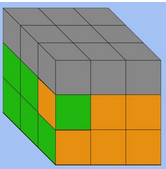If there are no edges available then take one out with the algorithm and then put it back in (as shown in this picture)

Repeat this until you have all 4 edges in the right place

Step 6: orientate the last layer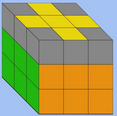Part 1: solve the cross

You need to learn this algorithm: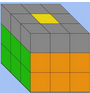If you have a dot as above:

Then do the algorithm once and you will have a triangleIf you have a triangle as above:

Then do the algorithm twice, make sure to hold it in the position shown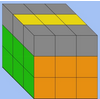If you have a line as above:

Then do the algorithm once, make sure to hold it in the position shown

Part 2: solve the corners
You need to learn this algorithm:Repeat this algorithm until the corners are solved, this may take some time, if you want to get faster you should learn the optimal way for each of the 7 possible cases.If you have 3 incorrect corners then hold the one correct corner in the front left position (as above).

Step 7: permute the last layer

Part 1: permute the cornersYou need to learn this algorithm: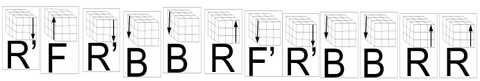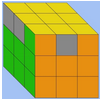if there are two correct corners next to each other, as above, then rotate them to the back and do the algorithmIf there are not two correct corners next to each other, as above, then do the algorithm and there will be

Part 2: permute the edges
You need to learn this algorithm: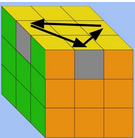This algorithm does the following cycle:

So if you have an edge correct, hold it at the back and do the algorithm once or twice

If you do not then do the algorithm once and you will.

Your 3x3x3 should now be solved.

What next?

The cubing community is a fast-growing worldwide community

The World Cube Association hosted 1154 cubing competitions in 2018. You can see if there are any near you at www.worldcubeassociation.org/competitions